• 子带自适应滤波器在进行自适应滤波之前将通过滤波器组将输入信号（远端信号及麦克风收集到的回声信号和近端噪声）进行分割并抽取，通俗来讲每个子带内的信号比较均匀，避免了全信号特征值较大容易发散的缺点，使得...

个人理解，仅供参考！

前面的仿真直接用外部输入信号实现的自适应算法虽然简单容易实现，但是当输入信号是相关性比较高的语音信号时，自适应算法的收敛速度会明显变得比较差。子带自适应滤波器在进行自适应滤波之前将通过滤波器组将输入信号（远端信号及麦克风收集到的回声信号和近端噪声）进行分割并抽取，通俗来讲每个子带内的信号比较均匀，避免了全带信号特征值较大容易发散的缺点，使得各个子带中进行自适应滤波的数据信号具有更低的相关性，从而加快了自适应滤波器的收敛速度。注意，自适应过程是在进行频带分割之后的信号块之间用自适应算法来进行每个频带之间的自适应迭代。

子带自适应滤波器的组成：分析滤波器、综合滤波器、自适应FIR滤波器

分析部分由分析滤波器和抽值滤波器组成，综合部分由综合滤波器和插值滤波器组成。分析滤波器和综合滤波器是根据设定好的参数直接进行设计。（这个过程有点复杂，我是用参考书配套代码做的仿真，如果也有同学需要的话可以留言~

数据处理过程：当数据输入到带有子带滤波器组的系统时，先由分析滤波器组进行频带分割，然后由抽值滤波器抽取后生成的子带信号传送个子带信号处理模块（在这里一般指的是自适应滤波器）进行信号处理，处理后的子带信号经过综合滤波器的插值和综合滤波器滤波后进行相加处理得到全带信号。子带滤波器的子带数、抽取或插值倍数的选择对滤波器的性能有很重要的影响。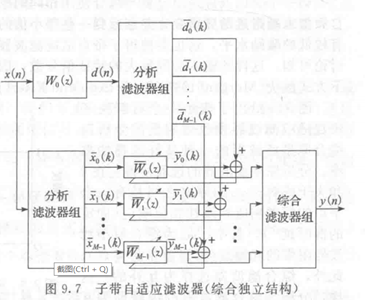展开全文• 自适应滤波算法labview源文件，是.VI格式的文件，labview2018或更低版本可以正常打开，可正常仿真labview
• 好不容易找到了该书的电子版，分享给大家学习使用，希望能够喜欢。AEC
• 获校级电子设计竞赛一等奖，基于STM32F407的FFT自适应滤波程序， 0—20KHz自适应滤波，应用Blackman_Win窗口减少幅值泄露，分辨率20Hz，滤波后频率误差100Hz，幅值误差100mv。欢迎批评指正
• 第 1 章分析了由于输入和输出滤波器组的原型滤波器的传统选择而导致的问题，并表明这个问题是不可避免的。 建议一种解决方法。matlab
• 针对欠定模型条件下定步长比例归一化子...该算法综合了子带自适应滤波、比例自适应算法及变步长方法的优点。仿真结果表明：与定步长比例归一化子带自适应滤波算法相比，所提算法具有更低的稳态误差和更快的收敛速度。
• 自适应滤波算法综述

万次阅读 多人点赞 2020-04-21 14:58:25
自适应滤波算法综述

绪论

• 自适应滤波是近30年以来发展起来的关于信号处理技术的方法。它是在维纳滤波、Kalman滤波等线性滤波基础上发展起来的一种最佳滤波方法。由于它具有更强的适应性和更优的滤波性能，从而在工程实际中，尤其在信息处理技术中得到了广泛的应用。
• 维纳滤波器等滤波器设计方法都是建立在信号特征先验知识基础上的。遗憾的是，在实际应用中常常无法得到信号特征先验知识，在这种情况下，自适应滤波器能够得到比较好的滤波性能。当输入信号的统计特性未知，或者输入信号的统计特性变化时，自适应滤波器能够自动地迭代调节自身的滤波器参数，以满足某种准则的要求，从而实现最优滤波。
• 自适应滤波的研究对象是具有不确定的系统或信息过程。这里的“不确定性”是指所研究的处理信息过程及其环境的数学模型不是完全确定的。其中包含一些未知因素和随机因素。
• 自适应滤波一般包括3个模块：滤波结构、性能判据和自适应算法。其中，自适应滤波算法的研究是自适应信号处理中最为活跃的研究课题之一，包括线性自适应算法和非线性自适应算法。非线性自适应算法具有更强的信号处理能力，但计算比较复杂，实际应用最多的仍然是线性自适应算法。

自适应滤波的基本原理自适应滤波算法

自适应滤波算法种类

最小均方误差算法（LMS）

• 由Widrow和Hoff提出的最小均方误差(LMS)算法，因其具有计算量小、易于实现等优点而在实践中被广泛采用。
• 迭代公式如下：
e ( n ) = d ( n ) − X ⃗ T ( n ) W ⃗ ( n ) W ⃗ ( n + 1 ) = W ⃗ ( n ) + 2 u e ( n ) X ( n ) e(n) =d(n)-\vec{X}^T(n)\vec{W}(n)\\ \vec{W}(n+1)=\vec{W}(n)+2ue(n)X(n) 其中 u 是步长因子，LMS算法的收敛条件是 0 < u < 1 / λ m a x 0<u<1/\lambda_{max} λ m a x \lambda_{max} 是输入信号自相关矩阵的最大特征值。
• 初始收敛速度、时变系统跟踪能力及稳态失调是衡量自适应滤波算法优劣的三个最重要的技术指标。由于主输入端不可避免地存在干扰噪声，自适应滤波算法将产生参数失调噪声。干扰噪声v(n)越大，则引起的失调噪声就越大。减小步长因子u可减小自适应滤波算法的稳态失调噪声，提高算法的收敛精度。然而步长因子u的减小将降低算法的收敛速度和跟踪速度。因此，固定步长的自适应滤波算法在收敛速度、时变系统跟踪速度与收敛精度方面对算法调整步长因子u的要求是相互矛盾的。为了克服这一矛盾，人们提出了许多变步长自适应滤波算法。R.D.Gitlin曾提出了一种变步长自适应滤波算法。其步长因子u(n)随迭代次数的增加而逐渐减小。Yasukawa提出了使步长因子u正比于误差信号e(n)的大小。而Gitlin等提出了一种时间平均估值梯度的自适应滤波算法。
• 变步长自适应滤波算法的步长调整原则是在初始收敛阶段或未知系统参数发生变化时，步长应比较大，以便有较快的收敛速度和对时变系统的跟踪速度；而在算法收敛后，不管主输入端干扰信号v(n)有多大，都应保持很小的调整步长以达到很小的稳态失调噪声。根据这一步长调整原则，有Sigmoid函数变步长LMS算法(SVSLMS)，其变步长u是e(n)的Sigmoid函数：
u ( n ) = β ( 1 1 + e − α ∣ e ( n ) ∣ ) u(n)=\beta(\frac{1}{1+e^{-\alpha|e(n)|}}) 该算法能同时获得较快的收敛速度、跟踪速度和较小的稳态误差。然而，该Sigmoid函数过于复杂，且在误差e(n)接近零处变化太大。不具有缓慢变化的特性，使得SVSLMS算法在自适应稳态阶段仍有较大的步长变化，这是该算法的不足。

递推最小二乘算法（RLS）

• LMS算法的优点是结构简单，鲁棒性强，其缺点是收敛速度慢。
• 基于最小二乘准则，RLS算法决定自适应滤波器的权系数向量W(n)使估计误差的加权平方和 J ( n ) = ∑ i = 0 n λ n − i ⋅ ∣ e ( i ) ∣ 2 J(n)=∑_{i=0}^nλ^{n-i}·|e(i)|^2 最小。
• RLS算法对输入信号的自相关矩阵 R x x ( n ) R_{xx}(n) 的逆进行递推估计更新，收敛速度快，其收敛性能与输入信号的频谱特性无关。
• 推导与上面有一定的相似性，定义加权自相关: R x x ( n ) = E [ λ n − i X ⃗ X ⃗ T ] R_{xx}(n)=E[\lambda^{n-i}\vec{X}\vec{X}^T] 可以得出RLS算法的基本关系：
W ⃗ ( n ) = W ⃗ ( n − 1 ) + k ( n ) e ( n − 1 ) \vec{W}(n)=\vec{W}(n-1)+k(n)e(n-1)
其中 k ( n ) = P ( n − 1 ) X ⃗ ( n ) λ + X ⃗ T ( n ) P ( n − 1 ) X ⃗ ( n ) , P ( n − 1 ) = R x x − 1 ( n ) k(n)=\frac{P(n-1)\vec{X}(n)}{\lambda+\vec{X}^T(n)P(n-1)\vec{X}(n)},P(n-1)=R^{-1}_{xx}(n)• 但是，RLS算法的计算复杂度很高，所需的存储量极大，不利于适时实现；倘若被估计的自相关矩阵 R x x ( n ) R_{xx}(n) 的逆失去了正定特性，这还将引起算法发散。为了降低RLS算法的计算复杂度，并保留RLS算法收敛速度快的特点，产生了许多改进的RLS算法。如快速RLS(Fast RLS)算法，快速递推最小二乘格型(Fast Recursive Least Squares Lattice)算法等。这些算法的计算复杂度低于RLS算法，但它们都存在数值稳定性问题。
• 改进的RLS算法着重于用格型滤波器的RLS算法，快速RLS算法就是在RLS格型算法基础上得到的。格型滤波器与直接形式的FIR滤波器可以通过滤波器系数转换相互实现。格型参数称为反射系数，直接形式的FIR滤波器长度是固定的，一旦长度改变则会导致一组新的滤波器系数，而新的滤波器系数与旧的滤波器系数是完全不同的。而格型滤波器是次序递推的，因此，它的级数的改变并不影响其它级的反射系数，这是格型滤波器的一大优点。RLS格型滤波器算法就是将最小二乘准则用于求解最佳前向预测器系数、最佳后向预测器系数，进行时间更新、阶次更新及联合过程估计。格型RLS算法的收敛速度基本上与常规RLS算法的收敛速度相同，因为二者都是在最小二乘的意义下求最佳。但格型RLS算法的计算复杂度高于常规RLS算法。格型RLS算法的数字精度比常规RLS算法的精度高，对舍入误差的不敏感性甚至优于LMS算法。

变换域自适应滤波算法

• 对于强相关的信号，LMS算法的收敛性能降低，这是由于LMS算法的收敛性能依赖于输入信号自相关矩阵的特征值发散程度。输入信号自相关矩阵的特征值发散程度越小，LMS算法的收敛性能越好。经过研究发现，对输入信号作某些正交变换后，输入信号自相关矩阵的特征值发散程度会变小。于是，Dentino等1979年首先提出了变换域自适应滤波的概念，其基本思想是把时域信号转变为变换域信号，在变换域中采用自适应算法。Narayan等对变换域自适应滤波算法作了全面的总结。
• 变换域自适应滤波算法的一般步骤是:
• 变换矩阵N*N的T矩阵，常用的正交变换有离散余弦变换(DCT)、离散傅立叶变换(DTFT)、离散Hartly变换及沃尔什—哈达玛（Walsh—Hadamard）变换等。变换后的信号为： X ′ = T ∗ X X^{'}=T*X
• 变换域的递推公式： W ( n + 1 ) = W ( n ) + 2 u e ( n ) P − 1 ( n ) X ( n ) W(n+1)=W(n)+2ue(n) P^{-1} (n)X(n) P ( n ) = d i a g [ P ( n , 0 ) , P ( n , 1 ) , … , P ( n , N − 1 ) ] P(n)=diag[P(n,0),P(n,1),…,P(n,N-1)] P ( n , l ) = β P ( n − 1 , l ) + ( 1 − β ) X T ( n , l ) ⋅ X ( n , l ) ， l = 0 , 1 … N − 1 P(n,l)=βP(n-1,l)+(1-β) X^T (n,l)·X(n,l) ，l=0,1…N-1 若令 Λ 2 = P ( n ) Λ^2=P(n) ，则权系数向量的迭代方程为：
W ( n + 1 ) = W ( n ) + 2 u e ( n ) Λ − 2 X ( n ) W(n+1)=W(n)+2ue(n)Λ^{-2} X(n)

仿射投影算法

• 仿射投影算法最早由K, Ozeki和T. Umeda提出，它是能量归一化最小均方误差(NLMS)算法的推广。仿射投影算法的性能介于LMS算法和RLS算法之间，其计算复杂度比RLS算法低。能量归一化最小均方误差(NLMS)算法是LMS算法的一种改进算法，NLMS算法可以看作是一种时变步长因子的LMS算法。其收敛性能对输入信号的能量变化不敏感。
• 仿射投影算法是NLMS算法的多维推广，假定P为投影阶数，仿射投影算法中权系数向量的修正量由下述方程组的最小二范解决定： Y ( k ) = X T ( k ) [ W ( k − 1 ) + Δ W ( k − 1 ) ] Y(k)=X^T (k)[W(k-1)+\Delta W(k-1)] 其中： Y ( k ) = [ y ( k ) , y ( k − 1 ) , … , y ( k − p + 1 ) ] Y(k)=[y(k),y(k-1),…,y(k-p+1)] X ( k ) = [ x ( k ) , x ( k − 1 ) , … , x ( k − p + 1 ) ] X(k)=[x(k),x(k-1),…,x(k-p+1)]
利用矩阵的广义逆可求得 Δ W ( k − 1 ) \Delta W(k-1) ，因此，仿射投影算法可表示为：
e ( k ) = Y ( k ) − X T ( k ) W ( k − 1 ) e(k)=Y(k)-X^T (k)W(k-1) g ( k ) = [ X T ( k ) X ( k ) + δ l ] − 1 e ( k ) g(k)=[X^T (k)X(k)+δl]^{-1}e(k) W ( k ) = W ( k − 1 ) + u Δ W ( k − 1 ) = W ( k − 1 ) + u X ( k ) g ( k ) W(k)=W(k-1)+u\Delta W(k-1)=W(k-1)+uX(k)g(k)

其他

• 共轭梯度算法
• 基于子带分解的自适应滤波算法
• 基于QR分解的自适应滤波算法
• 其他

其他不再具体说明。

自适应滤波算法性能评价

下面对各种类型的自适应滤波算法进行简单的总结分析。

• 变步长的自适应滤波算法虽然解决了收敛速度、时变系统跟踪速度与收敛精度方面对算法调整步长因子u的矛盾，但变步长中的其它参数的选取还需实验来确定，应用起来不太方便。
• 对RLS算法的各种改进，其目的均是保留RLS算法收敛速度快的特点而降低其计算复杂性。
• 变换域类算法亦是想通过做某些正交变换使输入信号自相关矩阵的特征值发散程度变小。提高收敛速度。
• 而仿射投影算法的性能介于LMS算法和RLS算法之间。
• 共轭梯度自适应滤波算法的提出是为了降低RLS类算法的杂性和克服某些快速RLS算法存在的数值稳定性问题。
• 信号的子带分解能降低输入信号的自相关矩阵的特征值发散程度，从而加快自适应滤波算法的收敛速度，同时便于并行处理，带来了一定的灵活性。
• 矩阵的QR分解具有良好的数值稳定性。

自适应滤波的Matlab仿真

正弦信号加噪的LMS自适应滤波

代码

clc,clear,close all;
g=100;
L=1024;%信号长度
k=128;%滤波器阶数
pp=zeros(g,L-k);
u=0.001;
for q=1:g
t=1:L;
a=1;
s=a*sin(0.05*pi*t);
figure(1);
subplot(311);
plot(s);
title('信号s时域波形');
xlabel('n');
axis([0,L,-a-1,a+1]);
xn=awgn(s,5);               %信噪比5dB的WGN
y=zeros(1,L);
y(1:k)=xn(1:k);
w=zeros(1,k);
e=zeros(1,L);
for i=(k+1):L
XN=xn((i-k+1):(i));
y(i)=w*XN';
e(i)=s(i)-y(i);
pp(i)=pp(i)+e(i);
w=w+u*e(i)*XN;
end
end
subplot(312)
plot(xn);
title('信号加高斯白噪声后的时域波形');
axis([0,L,-a-2,a+2]);
subplot(313)
plot(y);
axis([0,L,-a-1,a+1]);
title('LMS算法自适应滤波后的输出时域波形');

结果音频信号Rolling in the Deep的LMS自适应滤波

音频资源

已把音频放在了 https://pan.baidu.com/s/1L5vXa60c0wEATx2LQVLp-Q
提取码：vs7o

代码

1. LMSfilter.m
function [y,W,e]=LMSfilter(xn,d,L,mu)
% 输入参数:
%     xn   输入的信号序列      (列向量)
%     d   所期望的响应序列    (列向量)
%     L    滤波器的阶数        (标量)
%     mu   收敛因子(步长)      (标量)     要求0<mu<xn的相关矩阵最大特征值的倒数
% 输出参数:
%     W    滤波器的权值矩阵     (矩阵)
%          大小为M x t,
%     e   误差序列(t x 1)    (列向量)
%     y   实际输出序列         (列向量)
t = length(xn);
e = zeros(t,1);             % 误差序列,en(k)表示第k次迭代时预期输出与实际输入的误差
W = zeros(L,t);             % 每一行代表一个加权参量,每一列代表-次迭代,初始为0
% 迭代计算
for k = L:t                  % 第k次迭代
x = xn(k:-1:k-L+1);        % 滤波器L个抽头的输入
y = W(:,k-1).'*x;        % 滤波器的输出
e(k) = d(k)- y ;        % 第k次迭代的误差
W(:,k) = W(:,k-1) + 2*mu*e(k)*x;    % 滤波器权值计算的迭代式
end
% 求最优时滤波器的输出序列  r如果没有yn返回参数可以不要下面的
y = inf * ones(size(xn)); % inf 是无穷大的意思
for k = L:length(xn)
x = xn(k:-1:k-L+1);
y(k) = W(:,end).'* x;%用最后得到的最佳估计得到输出
end

1. main.m
clc;
clear all;
close all;

%% 产生信号源
[X,Fs] = audioread('Rolling in the Deep.wav');%音频过长无法计算自相关
signal = X(:,1); %取出双通道中其中一个通道作为信号源signal
audiowrite('原始音频.wav',signal,Fs); %创建原始音频.wav
n = length(signal);
t=(0:n-1);
figure(1);
subplot(3,1,1);
plot(t,signal);grid;ylim([-2 2]);
ylabel('幅度');
xlabel('时间');
title('原始音频信号');

%% 产生期望信号
dn = awgn(signal,10);             %加入信噪比为10dB的高斯白噪声
noise=dn-signal;
audiowrite('含噪音频.wav',dn,Fs); %创建含噪音频
subplot(3,1,2);
plot(t,dn);grid;ylim([-2 2]);
ylabel('幅度');
xlabel('时间');
title('含噪音频信号');

%% LMS滤波算法
M = 128;     %滤波器阶数M
u = 0.0004;  %滤波器的步长
[yLMS,W,eLMS] =LMSfilter(noise,dn,M,u);

%% 绘制去噪后的语音信号
subplot(3,1,3);
plot(t,eLMS);grid;ylim([-2 2]);
ylabel('幅度');
xlabel('时间');
title('去噪后的音频信号');
audiowrite('去噪音频.wav',eLMS,Fs);%保存去除噪声的音频

%%
e = signal-eLMS;%剩余噪声
figure(2);
subplot(2,1,1);
plot(t,e);grid;
ylabel('幅度');
xlabel('时间');
title('剩余的噪声');

%% 一小段三个信号比较
subplot(2,1,2);
t=(1000000:1005000);
plot(t,eLMS(1000000:1005000,1 ),'r',t,e(1000000:1005000,1),'g',t,signal(1000000:1005000,1),'b');
axis([1000000,1005000,-1,1]);
ylabel('幅度');
xlabel('时间');
legend('去噪后的语音信号','剩余噪声','原始音频');
title('一小段三个信号比较');

结果及分析

1. u=0.0004，SNR=10dB时：2. u=0.0008，SNR=10dB时：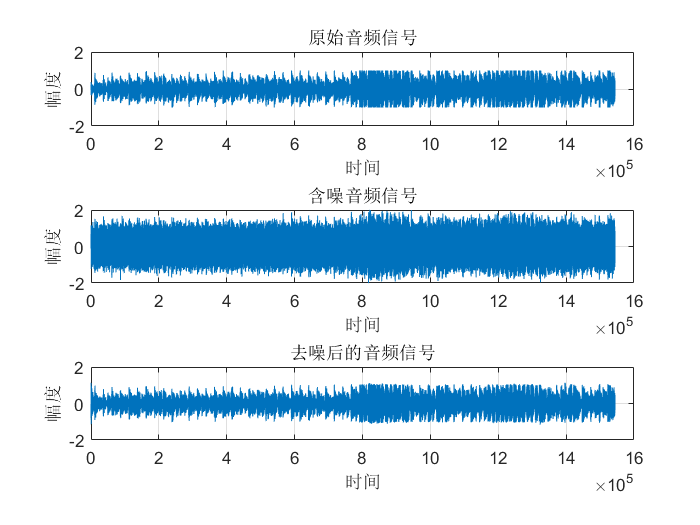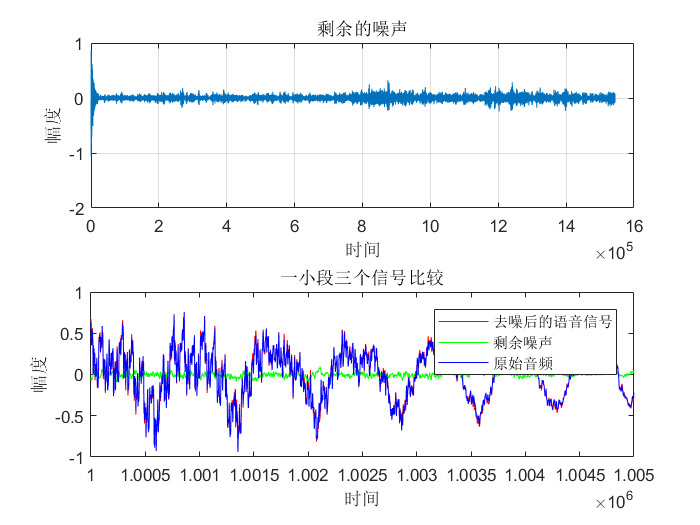3. u=0.0016，SNR=10dB时：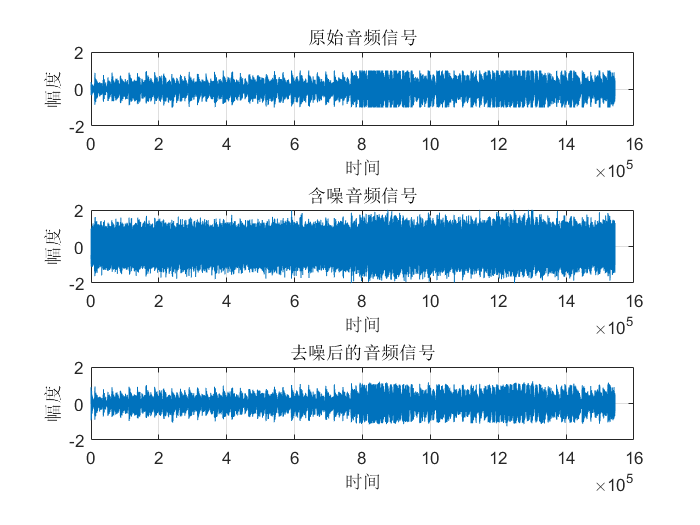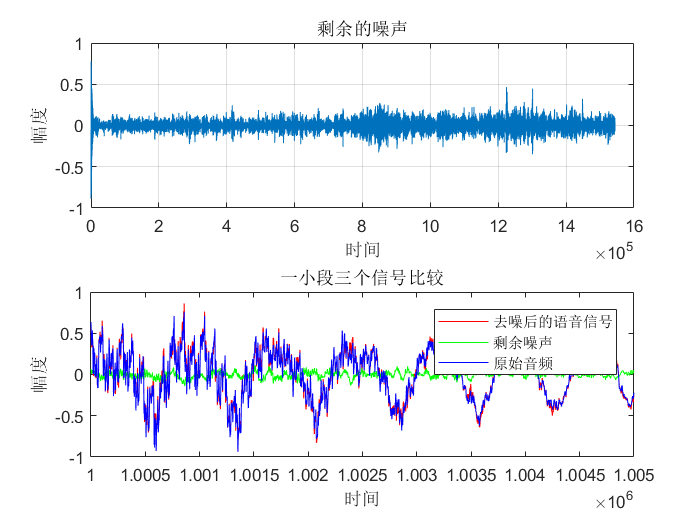4. u=0.0004，SNR=15dB时：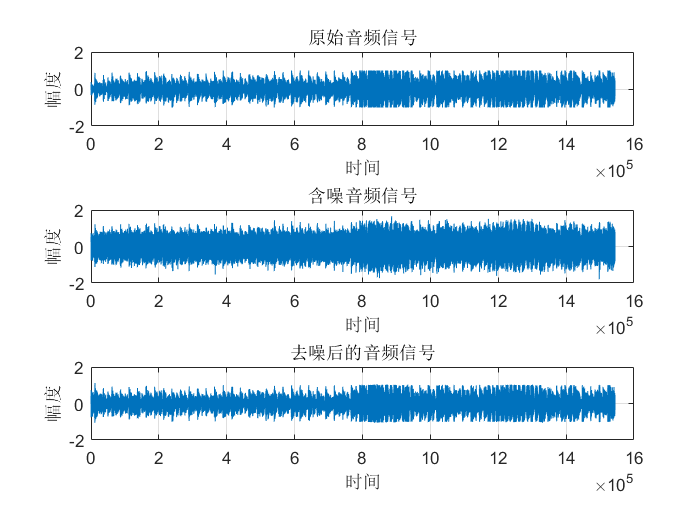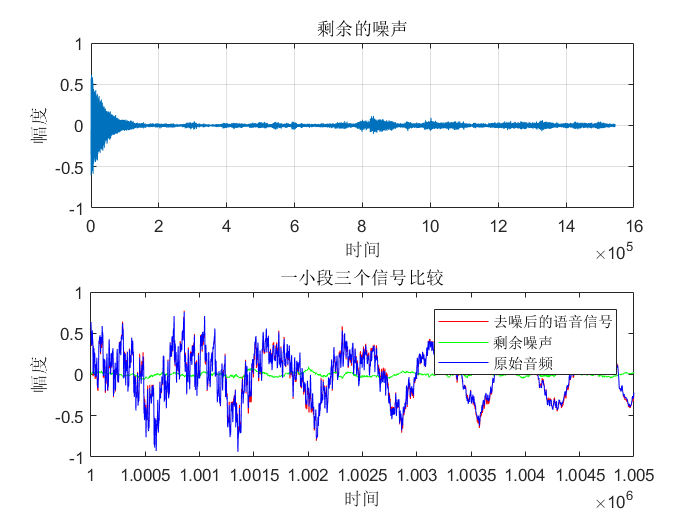• 从图中可以看出虽然真实的音频信号中混杂了很强的噪声，甚至噪声淹没了真实的信号，但是通过我们的LMS自适应滤波器后，可以很好的恢复出真实信号。所以自适应滤波器具有良好的去噪性能。
• 通过改变步长u，我发现随步长++，收敛时间–。但是当u增加，音频信号越来越清晰。但是当步长超过某一值时，音频中又开始很有噪声产生，再接着增加步长u，甚至会把音频信号也滤去掉。所以若 μ 值取的过小，收敛速度就会过于缓慢，当u取的过大时，又会造成系统收敛的不稳定，导致发散。
• 提高信噪比会使收敛时间变长。

其他

• 图像处理中wiener2()函数用于对图像进行自适应除噪滤波，其可以估计每个像素的局部均值与方差，调用方式如下：
J=wiener2(I,[M,N],noise);
%表示M*N大小邻域局部图像均值与偏差，采用像素自适应滤波器对图像I滤波

参考文献

1. https://wenku.baidu.com/view/457b0bc04028915f804dc241.html
3. https://blog.csdn.net/baidu_36161424/article/details/83244715
4. https://blog.csdn.net/zlh_hhhh/article/details/89061839
5. https://www.cnblogs.com/augustine0654/p/10041313.html
6. 耿妍，张端金.自适应滤波算法综述[J].信息与电子工程，2008，6(4)，315-320.
7. 邹艳碧, 高鹰.自适应滤波算法综述[J].广州大学学报(自然科学版)，2002，1(2)，44-48.
展开全文数字信号处理 信号处理
• 典教材“自适应滤波理论”（Simon Haykin）Matlab源代码! 内容简介 　在这本经典之作中，赫金（Haykin）教授系统全面地介绍了各种线性自适应滤波器和基于多层感知器的非线性自适应滤波器的基本原理与方法。在第四版...
• 自适应回声消除原理 　声学回声是指扬声器播出的声音在接受者听到的同时，也通过多种路径被麦克风拾取到。多路径反射的结果产生了不同延时的回声，包括直接回声和间接回声。 直接回声是指由扬声器播出的声音未经任何...

自适应回声消除原理

声学回声是指扬声器播出的声音在接受者听到的同时，也通过多种路径被麦克风拾取到。多路径反射的结果产生了不同延时的回声，包括直接回声和间接回声。

直接回声是指由扬声器播出的声音未经任何反射直接进入麦克风。这种回声的延时最短 ，它同远端说话者的语音能量，扬声器与麦克风之间的距离、角度 ,扬声器的播放音量，麦克风的拾取灵敏度等因素直接相关；

间接回声是指由扬声器播出的声音经过不同的路径 (如房屋或房屋内的任何物体 )的一次或多次反射后进入麦克风所产生的回声的集合。房屋内的任何物体的任何变动都会改变回声的通道。因此，这种回声的特点是多路径的、时变的。

自适应回声消除的基本思想是估计回音路径的特征参数，产生一个模拟的回音路径，得出模拟回音信号，从接收信号中减去该信号，实现回音抵消。其关键就是得到回声路径的冲击响应$\hat{h}(n)$，由于回音路径通常是未知的和时变的，所以一般采用自适应滤波器来模拟回音路径。自适应回音消除的显著特点是实时跟踪，实时性强。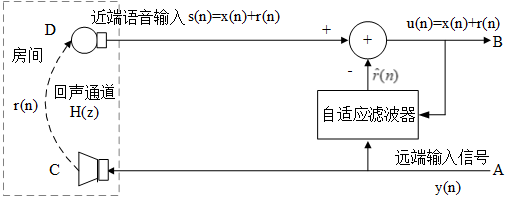回声消除原理框图

图中$y(n)$代表来自远端的信号 , $r(n)$是经过回声通道而产生的回声，$x(n)$是近端的语音信号。D端是近端麦克风，麦克风采集到的房间叠加的回声和近端说话人的语音。对回声消除器来说，接收到的远端信号作为一个参考信号，回声消除器根据参考信号由自适应滤波器产生回声的估计值$\hat{r}(n)$，将$\hat{r}(n)$从近端带有回声的语音信号减去，就得到近端传送出去的信号 。在理想情况下，经过回声消除器处理后，残留的回声误差$e(n)=r(n)-\hat{r}(n)$将为0，从而实现回音消除。

自适应滤波器算法的性能指标

收敛速度：滤波器的收敛速度越快越好，使正常通话开始后，通话者很快就感觉不到明显的回波存在。

稳态残留回波(稳定性)：即当滤波器收敛达到稳态后的回波输出量，实际中总是希望该参数越小越好。

算法复杂度：良好的算法应该在保持收敛速度的同时尽量降低计算复杂度，同时也能减少功耗

ITU-T G.168对各种回音抵消器产品在包括以上两个主要指标在内的各种指标规定了必须达到的标准。

自适应滤波器结构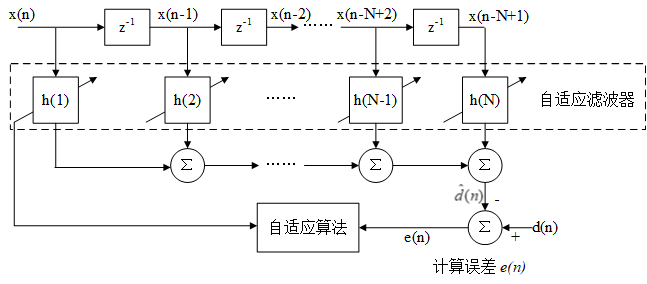自适应滤波器结构

图中所示滤波器的输入是$X(n)={x(n),x(n-1),...x(n-N+1)}^T$，$z^{-1}$滤波器z域模型的延迟单元(零状态)，滤波器的权重系数是$h(n)={h_1(n),h_2(n),...,h_N(n)}^T$，$d(n)$为期望输出信号，$\hat{d}(n)$为滤波器的实际输出，也称估计值，$\hat{d}(n)=\sum_{i=1}^Nx(n-i+1)h_i(n)$。$e(n)$是误差，$e(n)=d(n)-\hat{d(n)}$，由误差经过一定的自适应滤波算法来调整滤波系数，使得滤波器的实际输出接近期望输出信号。

传统的IIR和FIR滤波器在处理输入信号的过程中滤波器的参数固定，当环境发生变化时，滤波器无法实现原先设定的目标。自适应滤波器能够根据自身的状态和环境变化调整滤波器的权重。

自适应滤波器类型。可以分为两大类：非线性自适应滤波器线性自适应滤波器。非线性自适应滤波器包括基于神经网络的自适应滤波器及Volterra滤波器。非线性自适应滤波器信号处理能力更强，但计算复杂度较高。所以实践中，线性自适应滤波器使用较多

主要分为两类FIR滤波器、IIR滤波器。

1. FIR滤波器时非递归系统，即当前输出样本仅是过去和现在输入样本的函数，其系统冲激响应h(n)是一个有限长序列。具有很好的线性相位，无相位失真，稳定性较好
2. IIR滤波器时递归系统，即当前输出样本是过去输出和过去输入样本的函数，其系统冲激h(n)是一个无限长序列。IIR系统的相频特性是非线性的，稳定性不能保证。好处是实现阶数较低，计算量较少

由于IIR存在稳定性问题，因此一般采用FIR。

自适应滤波器算法按照不同的优化准则，常见自适应滤波算法有：递推最小二乘算法(RLS)，最小均方误差算法(LMS)，归一化均方误差算法(NLMS)，快速精确最小均方误差算法，子带滤波，频域的自适应滤波等等。

全带自适应稀疏算法

谱减法

本文研究的背景噪声是以汽车噪声和风声为主要对象，此类噪声的特点为加性的、局部平稳的、且与语音信号统计独立。谱减法根据噪声的加性特点，通过噪声能量估计和增益计算得到噪声的功率谱，然后从带噪语音的功率谱中减去估计出的噪声得到较为纯净的语音。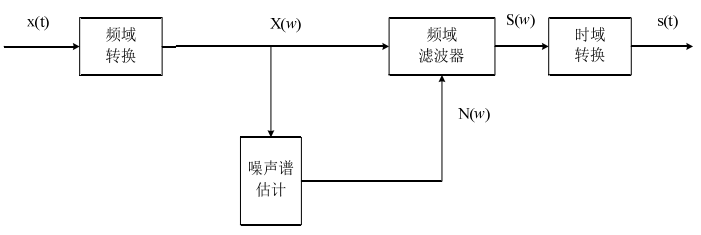由于谱减法没有考虑人耳听觉对语音频谱分布的幅度较为敏感的特点。因此采用谱减法进行滤波后，会给原信号带来噪声，使语音质量变差，并且会影响到其他处理，如语音编码等。用实际的语音信号检测谱减法的滤波效果，如图下所示，可以看出，经过谱减法后噪声得到了一定的消除，但频率较低的信号处滤波效果并不是很理想。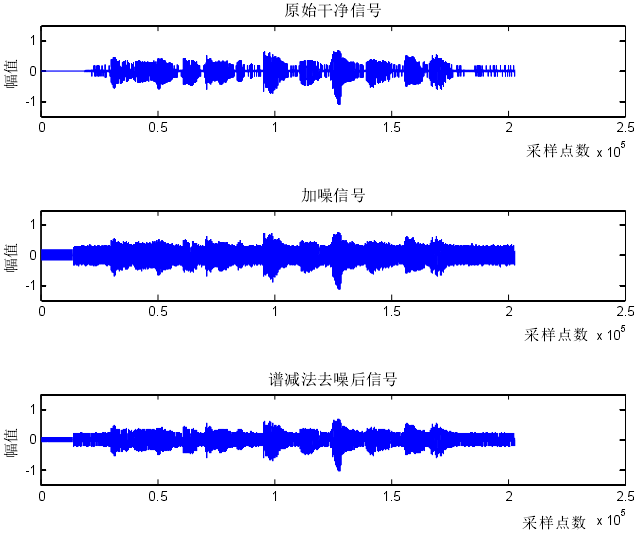递归最小二乘法 RLS

RLS算法的基本方法为：

$$\begin{array}{l}\mathop d\limits (n) = X_{}^T(n)H(n - 1)\\e(n) = d(n) - \mathop d\limits(n)\\k(n) = \frac{{P(n - 1)X_{}^3(n)}}{{\lambda + X_{}^T(n)P(n - 1)X_{}^3(n)}}\\P(n) = \frac{1}{\lambda }[P(n - 1) - K(n)X_{}^T(n)P(n - 1)]\\H(n) = H(n - 1) + K(n)e(n)\end{array}$$

K(n) 称为Kalman 增益向量，$\lambda$是一个加权因子，其取值范围$0<\lambda<1$，该算法的初始化一般令$H(-1) = 0$及$P(-1) = \frac{1}{\delta I}$，其中δ是小的正数。

最小均方算法 LMS

1959年由Widrow和Hoff提出了最小均方(Least Mean Square，LMS)算法，基于维纳滤波理论，采用瞬时值估计梯度矢量的算法，通过最小化误差信号的能量来更新自适应滤波器权值系数。

$\nabla (n) = \frac{{\partial [\mathop e\nolimits^2 (n)]}}{{\partial {\bf{h}}(n)}} = - 2e(n){\bf{X}}(n)$

按照自适应滤波器滤波系数矢量的变化与梯度矢量估计的方向之间的关系，可以写出LMS算法调整滤波器系数的公式如下所示：

$${\bf{h}}(n+1)={\bf{h}}(n)+\frac{1}{2}\mu [-\nabla (n)]\\={\bf{h}}(n)+\mu e(n){\bf{X}}(n)$$

上式中的$\mu$为步长因子。$\mu$值越大，算法收敛越快，但稳态误差也越大；$\mu$值越小，算法收敛越慢，但稳态误差也越小。为保证算法稳态收敛，应使$\mu$在以下范围取值：

$$0 < \mu < \frac{2}{{\sum\limits_{i = 1}^N {x(i)_{}^2} }}$$

从收敛速度来看，RLS 算法明显优于LMS 算法,但RLS 算法在运算上却比LMS 算法复杂得多,为了减小计算复杂度,并保留RLS 的收敛性能,人们提出了一些改进的RLS 算法。如RLS 格型算法,快速RLS 算法,梯度格型算法,快速横向滤波器算法等。总的来看,这些以收敛法都是以运算速度换取运算复杂性。

于是人们研究介于两者之间的一种算法, 如共轭梯度法、自仿射投影算法 等。共轭梯度法不需要RLS 中的矩阵运算,也没有某些快速RLS 算法存在的不稳定问题,但它的缺点是稳态误差比较大。

而LMS 算法的优点是运算简便,但它只有一个可调整参数,即步长因子μ ,可以用来控制收敛速率, 由于μ 的选择受系统稳定性的限制, 因此, 算法的收敛速度受到很大限制。为了加快收敛速度人们提出许多改进的LMS 算法。

• 优点：抑制旁瓣效应
• 缺点：LMS算法计算复杂度不高，但是其收敛速率较慢，并且随着滤波器阶数(步长参数)升高，系统的稳定性下降，要保证采用最小的步长参数，保证最小的失调，可能无法满足收敛标准

块处理LMS算法(BLMS)

为了对付LMS运算量大的问题，在LMS基础上提出了块处理LMS(BLMS)。它与LMS算法不同的是：LMS算法是每来一个采样点就调整一次滤波器权值；而BLMS算法是每K采样点才对滤波器的权值更新一次。这样BLMS算法的运算量就比LMS的运算量要小的多，但它的收敛速度却与LMS算法相同。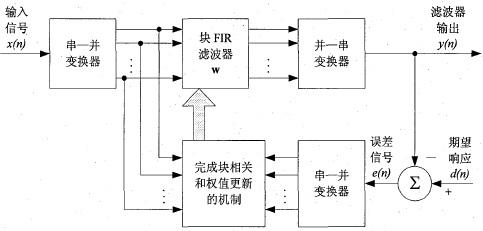块自适应滤波器的原理图

输入数据序列经过串一并变换器被分成若干个长度为L的数据块。然后将这些数据块一次一块地送入长度为M的滤波器中，在收集到每一块数据样值后，进行滤波器抽头权值的更新，使得滤波器的自适应过程一块一块地进行，而不是像时域传统自适应滤波算法那样一个样值一个样值地进行。

具体算法如下：

$$公式一：{\bf{h}}(n+1)={\bf{h}}(n)+\frac{1}{2}\mu [-\nabla (n)]={\bf{h}}(n)+\mu e(n){\bf{X}}(n)$$

由上面公式可知，可以推出：

$${\bf{h}}(n) = {\bf{h}}(n - 1) + \mu e(n - 1){\bf{X}}(n - 1)$$

代入公式一得：

$${\bf{h}}(n + 1) = {\bf{h}}(n - 1) + \mu e(n){\bf{X}}(n) + \mu e(n - 1){\bf{X}}(n - 1)$$

由于在块自适应滤波算法中，误差信号随抽样速率而变，故可得出对于每一个数据块，都有不同的用于自适应过程的误差信号值。因此，对于第k个数据块，可以对所有的可能值求乘积$x(kL+i)e(kL+i)$一次类推可得：

$$h(n+k+1)=h(n)+\mu e(n+kL)X(n+kL)+...+\mu e(n+kL+L)x(n+kL+L)=h(n)+\mu\sum_{i=0}^{L-1}e(n+kL+i)x(n+kL+i)$$

其中，$\\mu$为步长因子。

块自适应滤波算法具有与时域传统自适应滤波算法相似的特性。块LMS自适应滤波算法使用了更精确的梯度向量估计。

归一化最小均方算法 NLMS

归一化最小均方(Normalized Least Mean Squares，NLMS)算法是改进的LMS算法，根据原LMS算法中误差信号与远端输入信号的乘积，对远端输入信号的平方(功率)进行归一化处理，将固定步长因子的LMS算法变为根据输入信号时变的变步长NLMS算法，具体算法如下：

$$\begin{array}{l}estimated\_echo(i) = \sum\limits_{k = 0}^{N - 1} {{a_k}y(i - k)} \\{a_k}(i + 1) = {a_k}(i) + \frac{{{\beta _1}}}{{{P_y}(i)}}e(i)y(i - k)\\{P_y}(i) = (average(\left| {y(i)} \right|))_{}^2\end{array}$$

其中$a(k)$为滤波器的系数，$e(n)$为误差信号，$\beta_1$为固定环路增益，N为滤波器系数，$P_y(i)$为参考信号的能量估计。

• 优点：改善了LMS算法收敛速度慢的缺点。计算简单、更高的精度
• 缺点：输入相关信号时，收敛速率明显下降

后续研发除了归一化块处理LMS(BNLMS)：结合以上NLMS和BLMS两者的特点则有归一化块处理LMS(BNLMS)

变步长LMS(VSS LMS)

针对μ 值, 人们研究了许多变步长LMS 算法(Variable Step-Size LMS)，一般是在滤波器工作的开始阶段采用较大的μ值，以加快收敛速度，而在后阶段采用较小的μ值，可以减小稳态误差。这类算法的关键是确定在整个过程中μ值如何变化或μ值在何种条件满足下才改变。

• 优点：收敛速度快，
• 缺点：算法的稳定性和跟踪能力上较易受输入噪声的影响

仿射投影算法

仿射投影滤波器是归一化 LMS 滤波器的推广。具体来说，归一化滤波器抽头 系 数 的 调 整 项$\frac{\tilde{\mu }x(n)e^*(n)}{||x(n)||^2+\delta }$被 更 复 杂 的$\tilde{\mu }A^H(n)(A(n)A^H(n)+\delta I)^{-1}e(n)$所代替，其中 I 是单位矩阵，$\delta$是一个小正常数。

我们将仿射投影滤波器的设计用约束最优化问题来描述：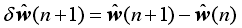其约束条件为：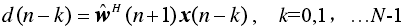式中 N 小于输入数据的维数 M。

当 N=1时，归一化 LMS 滤波器是这个约束最优准则的一个特例，其中仿射投影滤波器的阶数可看作 N 。

结合式上面两个公式基于多约束拉格朗日乘子法，建立如下代价函数：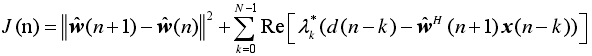引入如下定义：
1、 N*M 的数据矩阵 A(n) ，其共轭转置定义为：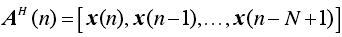2、 N *1的期望响应向量 d(n) ，其共轭转置定义为：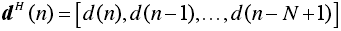3、 N *1的拉格朗日向量 $\lambda ^H$，其共轭转置定义为：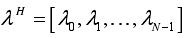利用式(3-12)重新定义代价函数为：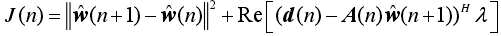对代价函数 J(n) 取权向量的微分可得：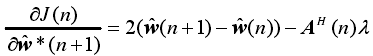设该导数为零，可得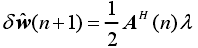为了从上式中消去拉格朗日乘子向量$\lambda$ ，首先利用式(3-13)和(3-14)的定义，重写
式(3-11)为：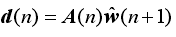然后，在式(3-18)两边同时左乘以 A(n) 并利用式(3-10)和式(3-19)消去权向量$\hat{w}$(n+1)，则得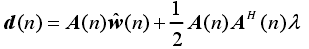由此，可以推出如下结果：

1、设 n 次迭代得到的数据$d(n)$与$A(n)\hat{w}(n)$之间的差为：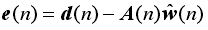2、矩阵乘积$A(n)A^H(n)$是一个 N *N 矩阵，它的逆为$(A(n)A^H(n))^{-1}$。

因此对式(3-20)求解向量$\lambda$，得：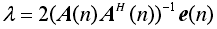将式(3-22)代入式(3-18)，得到的最优变化的权向量为：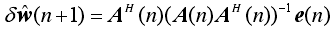最后，我们对需多次迭代的权向量进行控制。为此，把步长参数$\tilde{\mu}$代入式(3-23)，得到：等价地，可写出：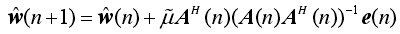式(3-25)为仿射投影滤波器的更新方程。因此仿射投影算法的运算过程描述如下：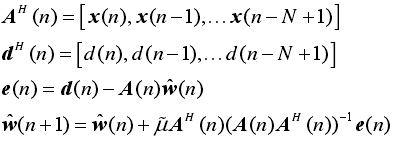其中 M 为抽头数，$\tilde{\mu}$为自适应常数，N 为滤波器阶数。

仿射投影算法较 NLMS 更适合处理输入信号具有相关性的情况，因为仿射投影算法滤波器系数的更新依赖于多个输入信号，而非只与上一时刻的输入信号相关。和 NLMS 算法类似，仿射投影算法的收敛速度也随着步长因子的变化而改变，因此仿射投影算法也可针对步长因子进行改进。通常情况下，仿射投影滤波器较归一化 LMS 滤波器收敛速度要快。然而，仿射投影算法性能的改善是以算法复杂度的增加为代价的，在实际应用中，对于要求实时性的场合会受到限制。

• 优点：收敛快
• 缺点：运算量很大

稀疏类自适应算法——PNLMS

通过对回声路径模型的分析，发现回声能量中较活跃系数均在时域聚集，且比重很小，其数值只有很少不为零的有效值，大多数都是零值或者接近零值，这就是回声路径具有的稀疏特性(基于时域对信号进行处理的一种特性)

PNLMS

根据回声路径的稀疏性，Duttweiler引入了比例自适应的思想，提出了比例归一化最小均方算法 PNLMS，按比例分配滤波器的权值向量大小，该算法对回声消除的发展具有非常重要的意义。

该算法采用与滤波器抽头稀疏成正比的可变步长参数来调整算法收敛速度，利用其抽头稀疏的比例值来判断当前权重稀疏所属的活跃状态，根据状态的不同，所分配的步长大小也有所差异，活跃抽头系数分配较大的步长参数，这样可以加速其收敛，而不活跃的抽头系数则相反，通过分配其较小的步长参数来提高算法的稳态误差。每个滤波器抽头被分别赋予了不同估计值，算法的稳态收敛性得到了明显改善。然而，PNLMS 有一个明显的缺点，即比例步长参数的选择引入了定值，这会导致估计误差累积，最后使算法在后期的收敛速度减慢下来。

优点：使算法对于稀疏的回声路径，在初始阶段拥有快速的收敛速率，与此同时降低了稳态误差，缺点

1. 由于 PNLMS 算法过分强调大系数的收敛，而当系数变小后，P 步长也随之变小，但随着算法的运行，则可能出现算法后期收敛速度慢、不能及时收敛的情况
2. 相比于NLMS算法增加了算法的复杂度
3. 另外在回声路径非稀疏情况下，收敛速度会变得比NLMS算法更慢

PNLMS++

PNLMS ++算法，在每个采样周期内，通过将NLMS算法和PNLMS算法之间进行交替来实现收敛速度方面的提升。但是PNLMS ++算法只在回声路径稀疏或高度非稀疏的情况下表现良好。

CPNLMS

CPNLMS 算法(Composite PNLMS)通过比较误差信号功率和已设阈值大小，来判断算法采用 PNLMS 还是 NLMS 算法

• 缺点：阈值的选取往往和实际环境有关，因此该算法在实际应用中不能通用

$\mu$准则MPNLMS

$\mu$准则MPNLMS算法：为了解决PNLMS算法后程收敛速度慢的问题，将最速下降法应用到PNLMS算法中，使用$\mu$准则来计算比例因子(使用对数函数代替PNLMS算法中的绝对值函数)

• 优点：使得滤波器权值向量的更新更加平衡，提升了PNLMS算法接近稳态时期的收敛速率
• 缺点：复杂性加大

改进比例归一化最小均方 IPNLMS

IPNLMS算法，基于L1范数将估计的回声路径权值向量均值与比例步长参数之和作为比例矩阵的对角元素(通过调整滤波器的比例参数步长)，使得IPNLMS有着和PNLMS相近的初始收敛速率，并且在非稀疏的回声路径条件下，IPNLMS的收敛速率相比PNLMS有所改善，但性能改善的同时也增加了计算复杂度。

改进的IPNLMS

在PNLMS类算法进行自适应迭代更新过程中，大抽头权值向量拥有大步长因子，这样提升了收敛速率，但滤波器自适应收敛至接近稳态时，大抽头权值向量将会产生较大的稳态误差。为了解决这一问题，P.A.Naylor提出了改进的IPNLMS(Improved IPNLMS，IIPNLMS)算法。IIPNLMS算法在IPNLMS算法的基础之上，对于数值较大的权值向量，使用较小的参数使得其步长成比例减小，从而降低系数噪声。

MPNLMS

MPNLMS算法引入最优步长控制矩阵，均衡了滤波器大小系数之间的更新，MPNLMS 中 P 步长的计算方式克服了 PNLMS 过分注重大系数收敛忽略小系数收敛的缺点，修正了 PNLMS 算法收敛后期速度慢的缺点，但

• 缺点：MPNLMS算法的运算过程中包含对数的计算，因此算法的运算复杂度相对较高。

SPNLMS

SPNLMS算法，将MPNLMS滤波器更新过程中的对数函数关系简为两段折线形式的形式。

SPNLMS的P步长计算函数$F(|w_l(k)|)$可以描述为：

$$F(|w_l(k)|)=\left\{\begin{matrix} 600*|w_l(k)|&&|w_l(k)\leq 0.005|\\ 3&&其他 \end{matrix}\right.$$

• 优点：相对于MPNLMS为减小运法复杂度
• 缺点：但收敛速度有所下降

改进的 MPNLMS算法，采用多个分段函数来近似 MPNLMS 的对数函数，从而降低算法的复杂度。

改进的 SPNLMS算法，通过控制步长控制矩阵迭代的频率降低算法复杂度。收敛速度也所下降

以上对 MPNLMS 的改进算法在减小算法运算复杂度的情况下损害了收敛性能和稳定性能。

稀疏控制(Sparse Control, SC)

有时候回声路径的稀疏程度会根据温度、压力以及房间墙面的吸声系数等等因素而产生变化，这就需要一种能够适应不同稀疏度变化的AEC算法。

稀疏控制比例回声消除算法(SC-PNLMSSC-MPLNMSSC-IPNLMS)，使用新的稀疏控制方法动态地适应回声路径的稀疏程度，使得算法在稀疏和非稀疏的回声路径条件下都有良好的性能表现，体现了稀疏控制类的自适应滤波算法能够提高算法对回声路径稀疏程度的鲁棒性。

小总结：通过上述对 PNLMS 算法的分析，可知导致 PNLMS 算法整体收敛速度慢的原因主要是大系数和小系数之间收敛的不均衡。尽管很多学者针对此缺陷提出了修正算法，如 PNLMS++、CPNLMS 等，但是 PNLMS 忽略小系数收敛的缺陷并未从根本上得到改善，因此这些改进算法的效果不是十分理想。Deng通过对滤波器系数收敛过程的定量分析，在权系数更新过程中推导出最优步长的计算方式，提出一种改进的算法——MPNLMS 算法。MPNLMS 中 P 步长的计算方式克服了 PNLMS 过分注重大系数收敛忽略小系数收敛的缺点，修正了 PNLMS 收敛后期速度慢的缺陷。

一种新的改进型 PNLMS 算法，因 PNLMS 算法只注重大系数更新，忽视小系数收敛，致使算法在收敛后期速度下降，因此在 P 步长引入的同时必须注意小系数的更新。MPNLMS 算法通过建立 P 步长与当前滤波器权系数的函数关系，在一定程度上解决了 PNLMS 算法后期收敛速度慢的问题，但滤波过程包含了对数运算，不利于系统的实时实现。

​ 　　本文通过定量分析滤波过程，并考虑到大、小系数的收敛，建立了一种新的 P步长与当前滤波器系数之间的映射关系，降低了算法的运算复杂度。 该改进算法以 PNLMS 算法为基础，通过改变收敛过程来克服 PNLMS 算法的缺陷

子带自适应滤波器(SAF)

在声学回声消除应用中，远端输入语音信号的相关性较高，然而，传统的方法是基于“信号的无关性”假设的，传统的全带LMS 和NLMS 等计算复杂度低的随机梯度算法收敛速度明显下降。

远端语音信号相关性有两层含义：

• 时域上：它表征语音信号相关矩阵特征值的扩散度
• 频域上：它表征远端语音信号的频谱动态范围

子带自适应滤波器：SAF算法将相关信号通过滤波器组分割成近似无关的各个子带独立信号(子带分割)。然后对子带信号进行多速率抽取来获得采样信号，再进行信号的自适应处理。为研究子带自适应滤波器，首先需要了解多速率信号抽取系统和滤波器组。

多速率系统

用于子带自适应滤波器的多速率抽取系统有下采样上采样两种，主要通过抽取和插值方法来使系统获得不同采样率。输入信号经过 N 个滤波器分频后的总采样点数是原信号的 N 倍，大幅度提高的采样数增加了计算量。

滤波器组

信号子带分割通过滤波器组实现。滤波器组由分析滤波器综合滤波器共同组成。而滤波器组的实质是一系列带通滤波器。

分析滤波器组将数字信号分割后抽取成多个子带信号，经过信号处理后，综合滤波器组再对子带信号进行插值和滤波相加而恢复成原来的信号。

子带自适应算法结构

在传统的 SAF中，子带自适应算法都是以最小化子带误差信号为目标的，这样基于局部目标函数误差的最小化不一定是全局误差能量最小化。而分析滤波器组在子带切割和综合滤波器组重建全带信号时皆会引入时延，在AEC 应用中，这样的时延会使包含近端语音的全带误差信号传到远端，为了消除时延的影响，无延时子带闭环结构系统以全局误差能量最小化为约束条件来调整滤波器系数。最后，确保自适应滤波算法能够收敛到最佳的滤波器系数。

• 优点：改善了全带自适应滤波算法在相关信号条件下的收敛速率
• 缺点：
• 但其稳态误差由于输出时存在的混叠分量而显著升高
• 当采用正交镜像滤波器组时，虽然可以通过子带系统将混叠部分相互抵消掉，但在现实中却无法实现

子带自适应算法的后续发展

问题：针对SAF算法稳态误差较高的问题

解决：提出了 基于最小扰动原理提出了归一化的SAF(normalized SAF，NSAF)算法。

优点：由于SAF类算法固有的解相关特性，NSAF在处理相关输入信号时比全带的NLMS收敛速度快，而且计算成本与NLMS不相上下

近几年研究人员为了能够提升AEC算法的收敛性能和稳态性能，在NSAF的基础上结合全带自适应滤波算法的成比例理论，提出了几种改进的NSAF算法，例如不同形式的变步长因子NSAF以及变正则化参数NSAF。为了在识别稀疏回声路径时快速收敛，文献[22,23]将NLMS算法中的成比例思想以类比的方式融合到NSAF算法中，提出了比例NASF(proportionate NASF，PNSAF)算法和μ准则PNSAF(μ-law PNASF，MPNSAF)算法。

因为子带结构中存在混叠分量问题

1. Keermann于1988年利用采样滤波器组技术消除了混叠现象，但是此举增加了算法复杂度。
2. 相邻子带间留安全频带，缺点：引入了空白频带，降低了信号质量。
3. 重叠子滤波器补偿法，缺点：因为交叉项而增加了运算量，还降低了收敛速度。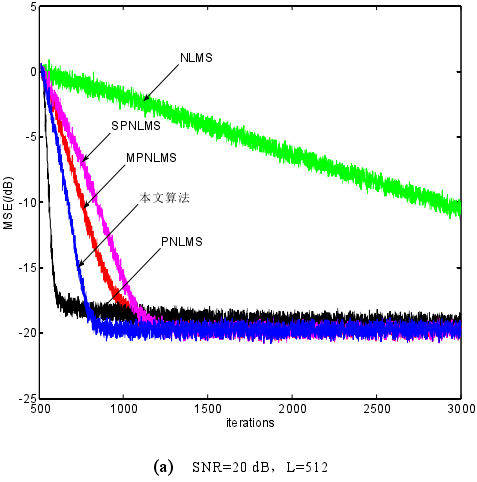2004年K. A. Lee 和 W. S. Gan提出了基于最小扰动原理的多带结构式自适应滤波器(Multiband Structured SAF，MSAF)算法，并给出了自适应滤波器抽头系数的更新方程。该结构完全不存在滤波器输出端的混叠分量问题。

多带自适应滤波器

子带自适应滤波器中每个子带单独使用一个子滤波器。该结构会导致输出端产生混叠分量，解决此问题的传统方法多以降低信号的质量或增大稳态误差为代价，Lee 和 Gan在 2004 年提出了一种全新多带结构。不同于子带滤波器在每个子带都使用不同的滤波器，多带结构的每个子带使用相同的全带滤波器，这很好地克服了输出端存在混叠分量的问题。

频域块LMS自适应滤波算法

块LMS自适应滤波算法中的线性卷积和线性相关均可以采用快速傅里叶变换(FFT)来实现。因此，块LMS自适应滤波算法的有效实现方法实际上是利用FFT算法在频域上完成滤波器系数的自适应。这样实现的块LMS自适应滤波算法称为频域块LMS自适应滤波算法(FDAF，Frequency-Domain Block Least MeanSquare Adaptive Filter)。

另一方面，数字信号处理中常用的重叠存储法和重叠相加法为快速卷积运算提供了强有力的工具，即利用离散傅里叶变换计算线性卷积。重叠存储方法是非自适应滤波两种方法中更为常用的一种方法。此外，值得注意的是，尽管滤波器能够以任意数量的重叠来实现，但当以50%重叠时运算效率达到最高。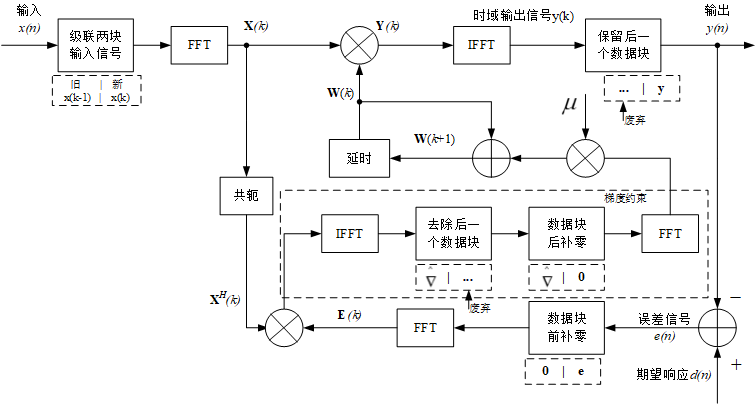基于重叠存储法的频域块LMS自适应滤波算法的信号流程图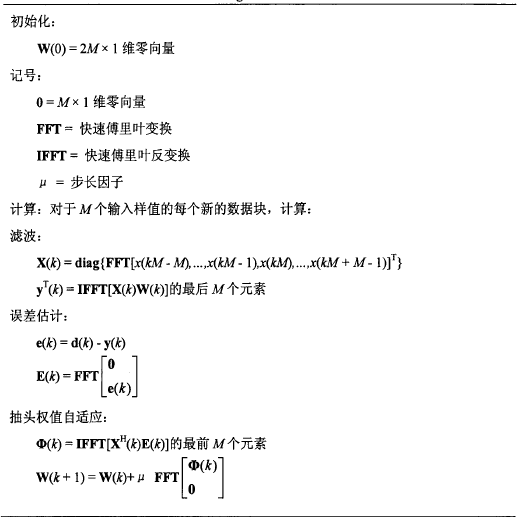问题：针对回声路径很长且复杂，并且回声延迟较高时，时域自适应滤波算法计算复杂度高的问题，

解决：提出了频域分块滤波(FDAF)算法，FDAF算法将长度为L的自适应滤波器分成FFT长度的整数倍个子块，对输入信号的每个子块进行频域内的LMS算法。

• 优点：当回声路径很长且复杂时计算量小，并且在收敛速度方面有略微提升。

相对于时域，FDAF具有许多优势。除了能够通过在频域中相乘来执行滤波器卷积之外，该变换还有效地减小了自适应滤波器的长度。因此，降低了自适应算法的计算复杂度。此外，为了降低计算复杂度，FDAF还可以提高收敛速度。这是由于滤波器更新中信号的自相关矩阵的特征值散布减小所导致的。

FDAF的这些优势最终需要权衡。FDAF的主要成本是增加的延迟和增加的内存需求。延迟成本来自需要通过频域滤波器延迟期望信号(或回声消除中的麦克风信号)。这导致在时域方法上增加了存储器存储，因为需要同时存储激励信号和期望信号。FDAF的早期方法使FFT的阶数与脉冲响应的大小大致相同。但是如前所述，回声消除等应用回波路径较长，会导致较大的延迟和存储需求。该缺点可以通过诸如多延迟自适应滤波器之类的方法来克服。在这种方法中，块大小可以小于所需的时域自适应滤波器，并且可以应用每个频点中的自适应滤波器来代替单个系数。因此，可以减轻FDAF的缺点，同时保持降低的计算复杂性和提高的收敛速度。

综上所述

《声学回声消除中子带和块稀疏自适应算法研究_魏丹丹》

远端输入语音信号的相关性较高，且声学回声信道的冲击响应一般只有少量的非零系数，因此是一个稀疏信道。

针对用于声学回声消除的子带和块稀疏算法进行了研究和改进，以达到提高算法跟踪性能和抗冲激鲁棒性的目的。本文的主要贡献如下：

首先，区别于传统文献中子带归一化自适应算法消除回声的方法，

我提出一种用于声学回声消除的新型子带归一化自适应滤波切换算法(LMS-NSAF)。

该算法核心思想是根据语音信号的状态不同，采用 VAD 快慢包络技术切换算法，当输入远端信号的瞬时能量值较大时，使用收敛速度快的子带 NLMS 算法，当输入信号的瞬时能量值较小时，则使用计算复杂度低的权重矢量更新公式，从而使得改进的子带 NLMS 算法在提高收敛性的同时又能降低算法的计算复杂度

基于多带结构的改进型自适应滤波切换算法NLMS-NSAF

首先远端语音信号利用包络法判别有无语音段，

然后将信号状态输出到自适应多带结构算法模块当中。

若语音区输入信号的短时能量较大，则使用收敛速度快的自适应滤波算法(NLMS)；

若语音区输入信号的短时能量较小，需考虑计算量低的算法(NSAF)，

当然，语音在无语音区时算法迭代停止。

对输入语音信号能量高低的判定是通过和阈值比较得到的。在充分考虑语音特性的情况下，切换算法实现了算法在收敛速度的优势，同时完成了同算法复杂度的优化选择。最后达到了提高滤波算法性能、降低运算量的目的。

回声路径是经典的稀疏路径，且语音信号作为远端输入时相关性较强，

总结

回声消除的挑战在于能否快速跟踪回声路径中的变化，同时又对大声干扰(例如双向通话)保持鲁棒性。这两个目标是矛盾的，因为为了快速适应回声路径的变化，系统需要具有快速收敛速度的自适应算法，这又意味着在出现双向通话时很容易发散。

在大多数系统中，实现了双向通话检测器以冻结自适应并防止发散。双向通话检测器的决策阈值通常需要对信号处理环境进行一些调整。这是一个不良的特性，因此，大多数系统都被调整为过于保守。换句话说，由于双向通话决策遗漏比双向通话虚假警报更重要，因此要进行调整以确保不会发生任何丢失的决策。这可能会导致系统产生高频率的错误警报，从而导致系统无法及时调整。

健壮的自适应算法在回声消除系统中的应用使双向通话检测器的保守性降低。由于大多数双向通话遗漏都发生在语音段的开始和偏移处，因此鲁棒的自适应算法试图使滤波器系数的自适应不受干扰，直到可以做出双向通话决定为止。由过去误差信号的电平调节的比例因子控制自适应算法的速率。因此，例如尚未收敛的系统将导致高比例因子。在双向通话的情况下，由于使用了过去的误差信号值，因此其开始会被延迟。

消除回声的两路径方法(AEC)论文进一步讨论了该主题。双向通话检测器的复杂性以及使用两组滤波器系数作为缓解快速收敛和双向通话问题的方法的目的变得更加清晰。双路径方法的问题是需要两个FIR操作，这给系统增加了内存和计算复杂度。鲁棒的自适应算法的应用可以帮助缓解过于保守的双向通话检测器的问题，而不会增加两径方法的负担。

除了居于核心地位的自适应滤波技术外，实际回音抵消技术应用系统中还包括远端信号检测、近端信号检测、舒适噪声产生、残留回波的非线性处理技术等，这些技术也有待改进。这样整个回音抵消器才能实现一个较好的回音抵消效果。

参考

声学回声消除中子带和块稀疏自适应算法研究_魏丹丹

《车载免提系统降噪算法的研究以及硬件实现》__张雪

频域块LMS自适应滤波算法的研究__田超

展开全文算法 机器学习 人工智能 深度学习 dwr
• 自适应滤波（回声消除）

千次阅读 2017-12-05 17:11:46
时域： ------------------------------------------------------------------------------------------------...又称为MDF, 与FBLMS,不同的是，h(n)也做了分块，分段块频域自适应滤波，处理长时回声，时延小

时域：

----------------------------------------------------------------------------------------------------------------------

LMS滤波器参数更新公式：权重更新收到输入信号的能量影响较大，输入信号能量越大，步长取值应该较小，保证不容易发散。

步长太大，容易发散。

步长太小，稳定，但是收敛速度慢，相互矛盾。

缺点：

1.逐个点更新，计算量大

2.瞬时跟踪能力较弱

NLMS滤波器

将输入信号的能量融合入权重更新公式，则优点：对于不太平稳的语音，相对LMS，也有较快的收敛性和平稳性。

计算量问题：

LMS算法：M阶自适应滤波器作用，有M次乘法，权重因子更新，则有M+1次乘法。M阶滤波器走一圈，则有2M+1次乘法。

NLMS算法：需要加上额外的能量乘法计算，则有3M+2次乘法。

计算量都正比于M阶系数。对于NLMS中能量的计算，可以用平均能量做平滑进行估计，减小计算量：AP算法：NLMS的一般化

利用了P列历史数据，后续的参数计算都是矩阵计算，不再是逐个点计算。有更好更快的收敛性，但是 计算量变大为重复利用过去的数据来提高收敛速度，但数据重用也导致了计算复杂度的增大。

当P为1时，退化成NLMS。RLS算法：最小化加权重的均方误差和。越久的点，则占的权重就越小，符合实际情况，满足非平稳的特性。

更新公式：其中，复杂度：----------------------------------------------------------------------------------------------------------------------

可变步长：----------------------------------------------------------------------------------------------------------------------

时延估计：

DTD检测：

http://www.docin.com/p-1263426732.html

http://www.docin.com/p-848898417.html&dpage=1&key=%E5%9B%9E%E9%9F%B3%E6%80%8E%E4%B9%88%E6%B2%BB

《一种新的回音消除的双向通话检测算法》

能量比较法

GEIGEL法    简单误判率高相关函数法   计算量大回声路径估计法   复杂

两矢量夹角法  就是下面那个公式如果v(n)由u(n)经过线性系统获得，则该系数为1，表示两者之间的线性关系，相关度。NLP后处理：

----------------------------------------------------------------------------------------------------------------------

子带自适应滤波器的若干问题

子带滤波器要完美重建需要满足一定条件，实际工程中，只需满足现实需求即可。

优点：

1.分成子带后，每个子带的信号变得相对平坦，频谱下采样后，有利于滤波器收敛。代码中，对子带频域信号进行循环偏移(时域抽取)，只取前面FS/D。

D决定了把频谱分成几个块，把这几个块进行循环右移动0~D-1，叠加在第一个块中。

Hz(i) 送入第几个子带信号，进行下采样(频谱下采样最后得到 W/D带宽的窄带信号)。

子带信号的时域和频域做法

子带时域，每隔D次更新      恢复，先通过FFT变换，变成频域子带信号(已经偏移好了)，之后如频域做法

频域做法就是偏移叠加，取第一个块。    恢复全带过程：  重新组合，   频域全带   Ifft    时域全带

相对于子带，过采样的好处，减少混叠。如果是严格下采样，相邻子带有混叠。

将子带信号进行频谱下采样后，可以重新排列，组合成全带信号。

因为子带 1,2,3 和 子带 7,6,5  共轭对称。所以仅需子带0,1,2,3,4可以恢复出全带信号。(i=1,2,...N/2-1)子带  与   N-i子带共轭对称。

2.分成子带后，滤波器的阶数较低，计算量较小。

3.分成子带后，有利于各个子带的并行处理。

缺点：

下采样会造成各个子带有混叠，而且混叠不可避免。对于单个子带来说，混叠相对于添加了噪声，这个会干扰自适应的过程。

为了减小混叠的影响，可以进行过采样，但是这会造成许多小的特征值(自相关矩阵)，这称为子带边界效应。

过采样的好处：减小混叠，均方误差较小；但是相对于严格下采样，一个快的初始收敛，慢的稳定收敛渐进线(边界效应，小的特征值)，如图：解决的思路：1.融合自适应cross-filter滤波器进行混叠补偿。 2.采用MSAF。 3.采用闭环结构。最小化全局的均方误差，而不是子带。

fir1(127,1/4)低通原型滤波器，Hi(z) = 原型滤波器  指数调制，为复数，所以频谱不会对称。四个子带； 过采样2倍，减小混叠，但是造成小的特征值；严格下采样，还是包含不希望的混叠分量。

cross-filter，相邻子带之间相互影响的因素考虑进去：如图开环结构：

对输入信号和期望信号都进行子带分解，每个子带滤波器都各自独立的进行自适应收敛，它不是全局均方误差最小化，而是

各个子带均分误差之和最小。闭环结构：

对输入信号和误差信号进行子带分解，最小化全局整频带的均方误差最小，有利于减小边界效应和混叠效应，合成时有时延对齐。PNLMS 加快收敛，误差较大

IPNLMS(improved proportionate) 扩展了NLMS算法，使用不同的步长，针对不同的系数，收敛率不依赖于信号的能量强度。

PMSAF 使用一系列子带信号 用全带滤波器

PMSAF在自适应阶段使用子带信号，这是与IPNLMS最主要的区别。

与之相似的是，步长的计算基于每个系数的幅度。

基本思想：刚开始用大步长，后面用小步长。梯度大时，用小步长，梯度小时，用大步长。

BLMS     分块的LMS时域算法

FBLMS   分块的LMS频域实现算法，可能造成大的时延

PFBLMS  分块频域快速块LMS，可以减小时延，处理长时回声

PBFDAF  或者说FBLMS是MDF的特殊情况，h(n)不作分块，或者说分块为1.

又称为MDF, 与FBLMS,不同的是，h(n)也做了分块，分段块频域自适应滤波，处理长时回声，时延小

展开全文• 自适应滤波实例之系统辨识

千次阅读 2020-07-28 12:38:49
实验模型 x(n)：随机信号，服从N(0,1)分布 h(n)：未知的系统响应（由5阶FIR低通滤波器模拟...设自适应滤波器的长度N=13，W0=[0 0 … 0 0] 选择步长，根据μ≤2NE[∣x(n)∣2]=213=0.1538\mu \le \frac{2}{NE[|x(n){{|}^{自适应滤波 系统辨识
• 程序 function [w,e,yn] = my_LMS(xn,dn) %% LMS实现程序 % 输入： % xn 输入信号 % dn 理想信号 % L 迭代次数 % k 滤波器的阶数 % 输出： % w 滤波器的系数矩阵 大小为 k×L 每一列代表一次迭代后的系数 % e...matlab
• 自适应滤波是近30年以来发展起来的关于信号处理技术的方法。它是在维纳滤波、Kalman滤波等线性滤波基础上发展起来的一种最佳滤波方法。由于它具有更强的适应性和更优的滤波性能，从而在工程实际中，尤其在信息处理...matlab 算法
• 自适应滤波实例之噪声抵消

千次阅读 2020-07-28 15:06:54
目标：d(n)中包含音乐信号s’(n)和白噪声v’(n)，v’(n)为v(n)经过系统h(n)后的输出，则x(n)=v(n)与v’(n)具有相关性，利用x(n)通过自适应滤波估计出v’(n)，从而抵消d(n)中的v’(n)，恢复出音乐信号。 d(n)=[s(n)+v...自适应滤波 matlab
• WebRtc AEC核心算法之一：频域自适应滤波 WebRtc和Speex作为目前开源的语音增强平台，可以给非科班出身的工程师一探究竟的途径，接下来以WebRtc的Aec模块，研究一下比较核心的算法和实现。一直以来在心目中AEC就是...
• 最小均方自适应滤波算法具有结构简单、性能稳定等优点，广泛应用于自适应噪声对消中，但其收敛速度与误差存在矛盾，不能同时得到满足。基于此，提出了改进的归一化最小均方自适应滤波算法，在计算输入信号功率时，...
• 自适应滤波去噪

千次阅读 2018-11-07 23:22:37
自适应滤波器具有在未知环境下良好的运作并跟踪输入统计量随时间变化的能力。尽管对于不同的应用有不同的实现结构，但是他们都有一个基本的特征：输入向量X(n)和期望响应d(n)被用来计算估计误差e(n)，即e(n)=d(n)-X...
• 设计了一种用于现代电子通信对抗的超低频信号自适应滤波系统，系统的硬件部分由XC2S100E-5PQG208C型芯片、信号处理模块、电源管理模块、自适应滤波模块、通信存储模块等部分构成；分别给出了系统主要硬件模块的电路...
• 自适应滤波-----LMS（Least Mean Square）算法

万次阅读 多人点赞 2018-10-05 17:26:50
自适应滤波的意义所在 自适应滤波器解决非平稳的过程，因为实际信号的统计特性可能是非平稳的或者是未知的。 自适应滤波器的特点： 1.没有关于待提取信息的先验统计知识 2.直接利用观测数据依据某种判据...自适应滤波 维纳滤波 最小均方误差
• 本文在论述自适应滤波的基本原理及其简单应用的基础上，简要介绍了LMS自适应滤波算法、RLS自适应滤波算法、变换域自适应滤波算法、仿射投影算法、共轭梯度算法、基于子带分解的自适应滤波算法、基于QR分解的自适应...
• 一、获取代码方式 获取代码方式1： 完整代码已上传我的资源：【心电信号】基于matlab自适应滤波算法胎儿心电信号提取【含Matlab源码 953期】 获取代码方式2： 通过订阅紫极神光博客付费专栏，凭支付凭证，私信博主，...matlab 算法 机器学习
• 在对仿射组合滤波器自适应算法性能分析的基础上，提出了一种新的组合参数更新公式，该更新公式中的固定系数改为与滤波器输出相关的函数，使仿射组合自适应滤波算法可以更好地实时跟踪滤波器的性能变化。...自适应算法
• 自适应滤波（LMS，RLS）

万次阅读 多人点赞 2016-06-26 11:00:29
自适应滤波存在于信号处理、控制、图像处理等许多不同领域，它是一种智能更有针对性的滤波方法，通常用于去噪。 图中x（j）表示 j 时刻的输入信号值，y（j）表示 j 时刻的输出信号值，d（j）表示 j 的参考信号值...自适应滤波器 最小均方误差 最小二乘
• 这次给了四个题目，分别实现了信息滤波、UD滤波、遗忘滤波和自适应滤波matlab 卡尔曼滤波算法
• 频域自适应滤波算法及应用本科毕业设计频域自适应滤波算法及应用华 南 理 工 大 学毕 业 设 计 (论文) 任 务 书兹发给 级 班学生毕业设计(论文)任务书，内容如下：1.毕业设计(论文)题目：频域自适应滤波算法及应用2....
• matlab代码用于自适应滤波和回波对消，代码采用LMS、RLS、DFT、子带滤波。
• 采用算数均值滤波器，几何均值滤波器，中值滤波自适应滤波器对图像进行滤波操作，并输出图像。 首先放一下结果： （1）算术均值滤波器 计算窗口的和并求平均 程序中对图像边缘不处理。右图为加入椒盐噪声，...OpenCV 算数均值滤波 几何均值滤波 中值滤波 自适应滤波
• 行业资料-电子功用-基于自适应滤波的伺服电机控制系统及力矩波动控制方法.pdf
• 基于对自适应滤波算法的研究可以得出，GSC（Generalized Sidelobe Canceller）框架是所有降秩滤波算法的统一基础模型，通过对GSC一般结构的降秩模型的研究。文中提出了3种能够改善一般结构结果的优化模型，PC......